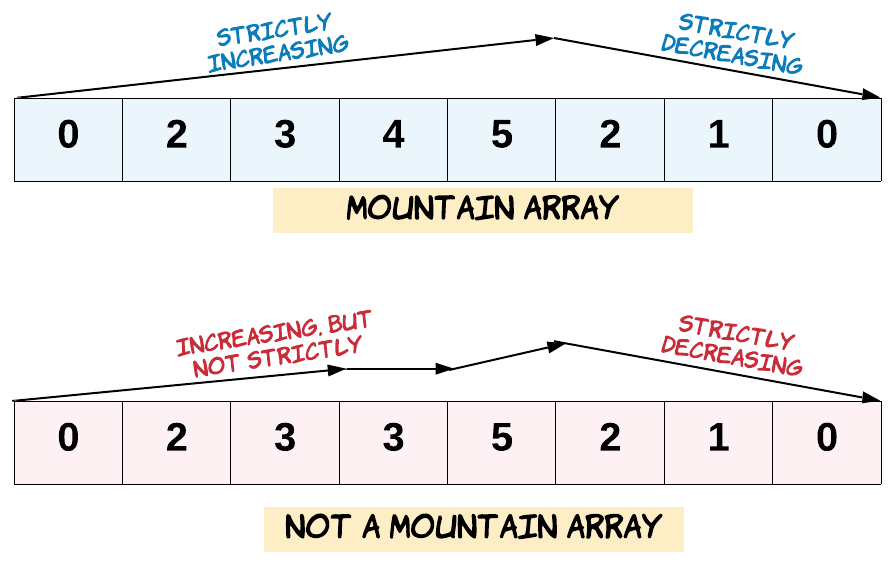# GeetCode Hub

Given an array of integers `arr`, return `true` if and only if it is a valid mountain array.

Recall that arr is a mountain array if and only if:

• `arr.length >= 3`
• There exists some `i` with `0 < i < arr.length - 1` such that:
• `arr < arr < ... < arr[i - 1] < arr[i] `
• `arr[i] > arr[i + 1] > ... > arr[arr.length - 1]`Example 1:

```Input: arr = [2,1]
Output: false
```

Example 2:

```Input: arr = [3,5,5]
Output: false
```

Example 3:

```Input: arr = [0,3,2,1]
Output: true
```

Constraints:

• `1 <= arr.length <= 104`
• `0 <= arr[i] <= 104`

class Solution { public boolean validMountainArray(int[] arr) { } }